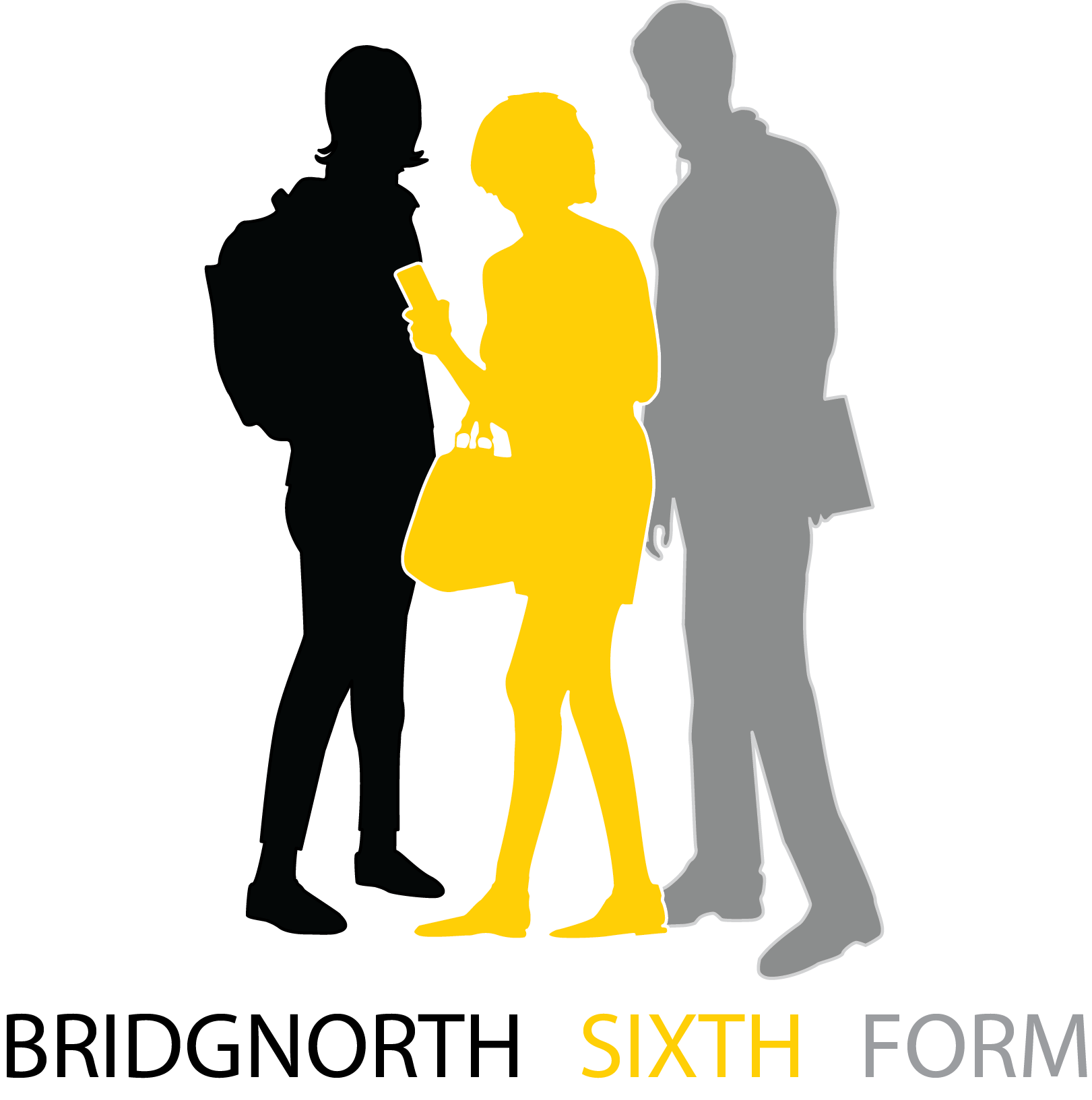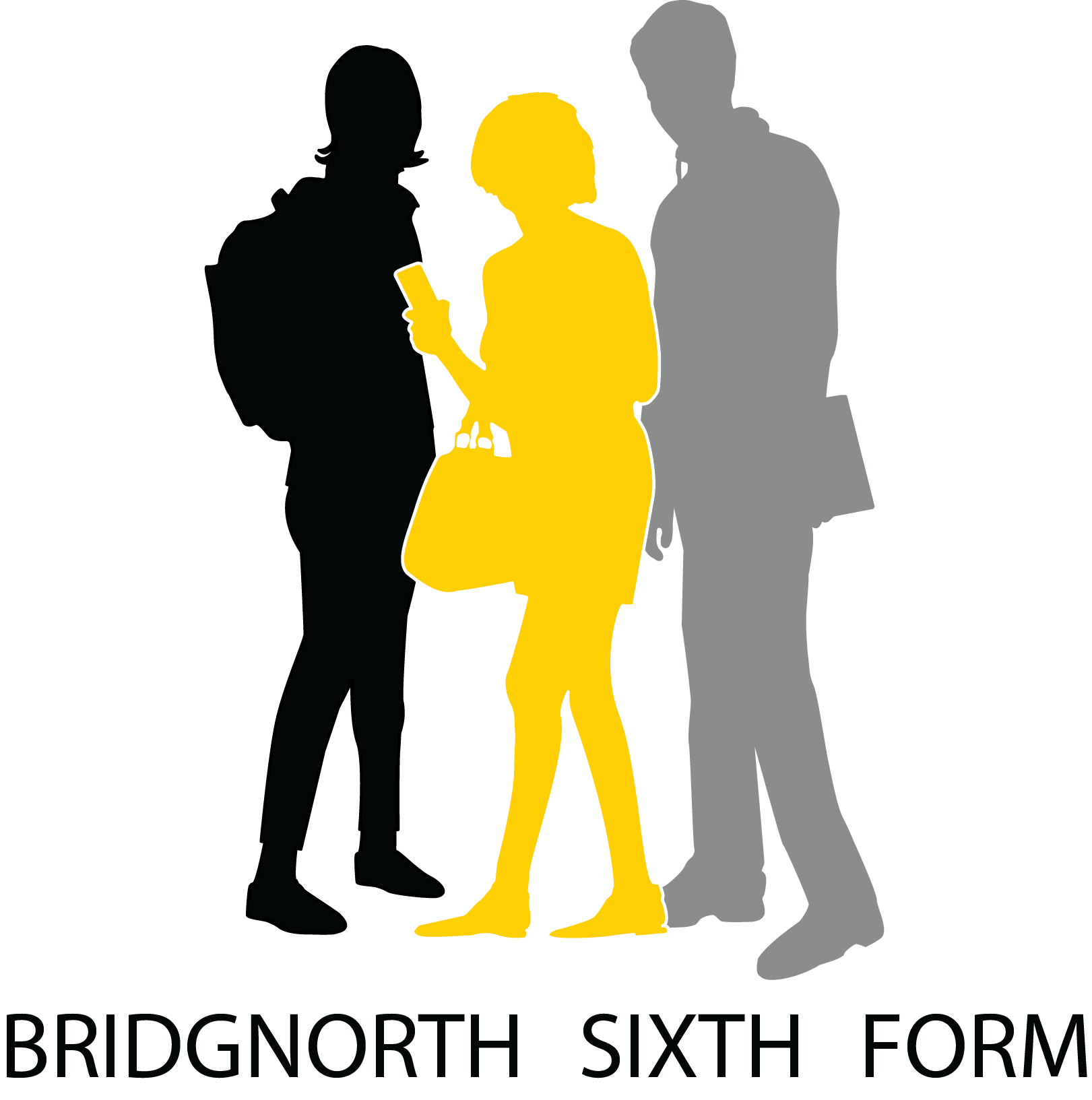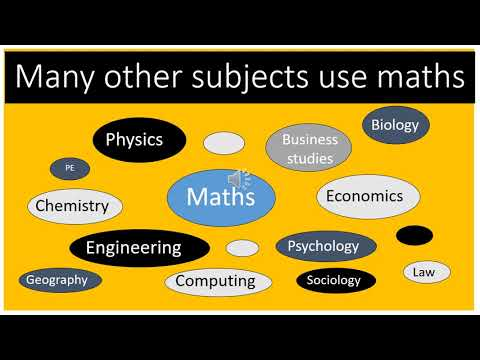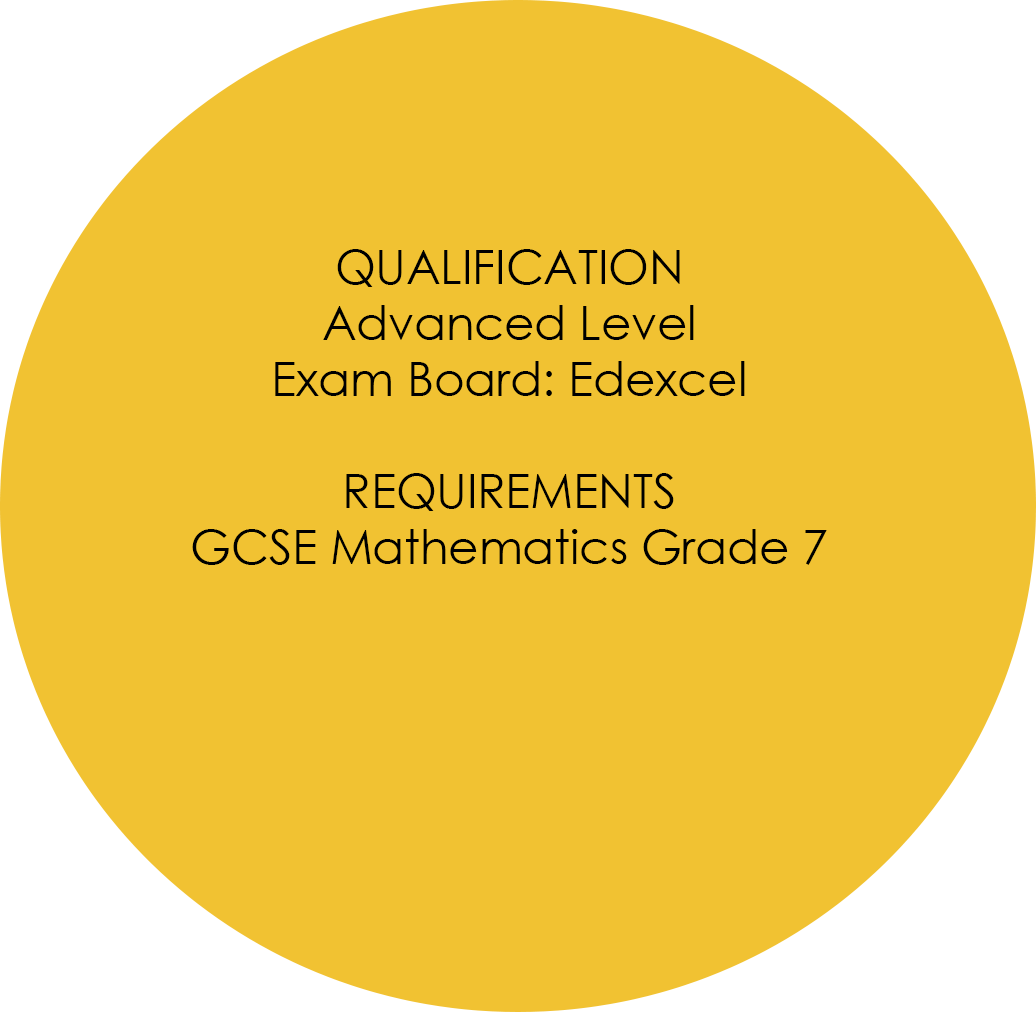#Bridgnorth Sixth Form @ Oldbury WellsCall: 01746 760505

## Maths### Why study Mathematics?

Mathematics is a versatile subject, respected by employers and universities. Mathematics students become better at thinking logically and analytically, and through problem solving develop resilience and are able to think creatively and strategically. Mathematics is a requirement for most Science and Engineering degree courses and the skills you learn in Mathematics are of great benefit in a range of other subjects such as Sciences, Computing and Business Studies.

### What skills are required?

A Level Mathematics requires students not only to extend their range of Mathematical skills and techniques, but also to be able to develop a greater understanding of how different fields of Mathematics are connected, to be able to apply Mathematics to other areas of study, to solve Mathematical problems in a variety of contexts and to construct Mathematical proofs. In order to do this, students need to have a secure and in-depth understanding of all areas of Mathematics from GCSE, which is why we ask for a grade 7 or above. It is also useful for A Level Mathematics students to have well developed problem solving skills.

### Course Content

Over the two year course students will cover the following content:

Pure Mathematics
Topic 1 - Proof
Topic 2 - Algebra and functions
Topic 3 - Coordinate geometry in the (x,y) plane
Topic 4 - Sequences and series
Topic 5 - Trigonometry
Topic 6 - Exponentials and algorithms
Topic 7 - Differentiation
Topic 8 - Integration
Topic 9 - Numerical methods
Topic 10 - Vectors

Applied Mathematics
Section A: Statistics
Topic 1 - Statistical sampling
Topic 2 - Data presentations and interpretation
Topic 3 - Probability
Topic 4 - Statistical distributions
Topic 5 - Statistical hypothesis testing
Section B: Mechanics
Topic 6 - Quantities and units in mechanics
Topic 7 - Kinematics
Topic 8 - Forces and Newton’s laws
Topic 9 - Moments

### How will it be assessed?

Paper 1: Pure Mathematics 1 Covering all Pure Mathematics topics

• 2 hours
• Calculator allowed
• 100 marks

Paper 2: Pure Mathematics 2 - Covering all Pure Mathematics topics

• 2 hours
• Calculator allowed
• 100 marks

Paper 3: Statistics and Mechanics Covering Statistics topics in Sec A and Mechanics in Sec B

• 2 hours
• Calculator allowed